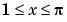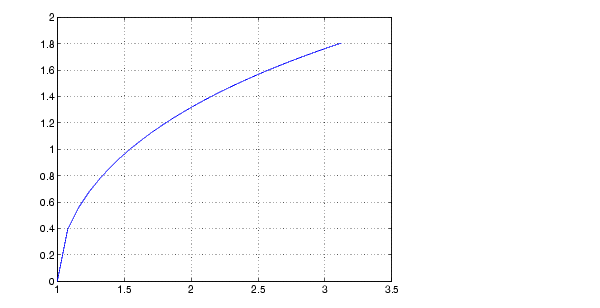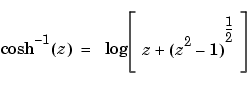MATLAB Function Referenceacosh

Inverse hyperbolic cosine

Syntax

• ```Y = acosh(X)
```

Description

```Y = acosh(X) ``` returns the inverse hyperbolic cosine for each element of `X`.

The `acosh` function operates element-wise on arrays. The function's domains and ranges include complex values. All angles are in radians.

Examples

Graph the inverse hyperbolic cosine function over the domain.

• ```x = 1:pi/40:pi;
plot(x,acosh(x)), grid on```

Definition

The hyperbolic inverse cosine can be defined as

•Algorithm

`acosh` uses FDLIBM, which was developed at SunSoft, a Sun Microsystems, Inc. business, by Kwok C. Ng, and others. For information about FDLIBM, see http://www.netlib.org.

See Also

`acos`, `cosh`

© 1994-2005 The MathWorks, Inc.